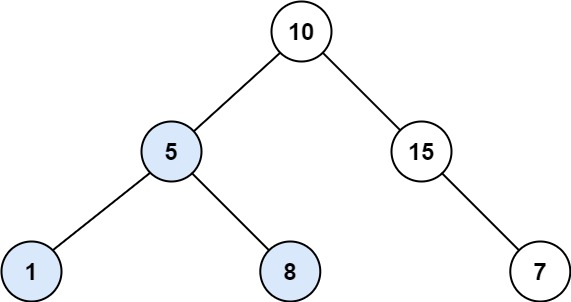## 333. Largest BST Subtree

Given the root of a binary tree, find the largest subtree, which is also a Binary Search Tree (BST), where the largest means subtree has the largest number of nodes.

A Binary Search Tree (BST) is a tree in which all the nodes follow the below-mentioned properties:

• The left subtree values are less than the value of their parent (root) node's value.
• The right subtree values are greater than the value of their parent (root) node's value.

Note: A subtree must include all of its descendants.

Follow up: Can you figure out ways to solve it with O(n) time complexity?

Example 1:```Input: root = [10,5,15,1,8,null,7]
Output: 3
Explanation: The Largest BST Subtree in this case is the highlighted one. The return value is the subtree's size, which is 3.```

Example 2:

```Input: root = [4,2,7,2,3,5,null,2,null,null,null,null,null,1]
Output: 2
```

Constraints:

• The number of nodes in the tree is in the range `[0, 104]`.
• `-104 <= Node.val <= 104`

## Rust Solution

``````struct Solution;
use rustgym_util::*;

trait Postorder {
fn postorder(&self, max_size: &mut usize) -> Option<(usize, i32, i32)>;
}

fn postorder(&self, max_size: &mut usize) -> Option<(usize, i32, i32)> {
if let Some(node) = self {
let node = node.borrow();
let val = node.val;
let mut size = 1;
let mut min = val;
let mut max = val;
let left = node.left.postorder(max_size);
let right = node.right.postorder(max_size);
if let Some(left) = left {
if left.2 < val {
size += left.0;
min = min.min(left.1);
} else {
return None;
}
} else {
return None;
}
if let Some(right) = right {
if val < right.1 {
size += right.0;
max = max.max(right.2);
} else {
return None;
}
} else {
return None;
}
*max_size = (*max_size).max(size);
Some((size, min, max))
} else {
Some((0, std::i32::MAX, std::i32::MIN))
}
}
}

impl Solution {
fn largest_bst_subtree(root: TreeLink) -> i32 {
let mut res = 0;
root.postorder(&mut res);
res as i32
}
}

#[test]
fn test() {
let root = tree!(10, tree!(5, tree!(1), tree!(8)), tree!(15, None, tree!(7)));
let res = 3;
assert_eq!(Solution::largest_bst_subtree(root), res);
let root = tree!(1, tree!(3, tree!(4), None), tree!(2, None, tree!(5)));
let res = 2;
assert_eq!(Solution::largest_bst_subtree(root), res);
let root = tree!(
4,
tree!(2, tree!(2, tree!(2, tree!(2), None), None), tree!(3)),
tree!(7, tree!(5), None)
);
let res = 2;
assert_eq!(Solution::largest_bst_subtree(root), res);
}
``````

Having problems with this solution? Click here to submit an issue on github.